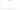# د.ب.‏

## Bahraini Dinar

Bahrain uses the dinar as its official currency. There are 1000 fils in it. The Bahraini dinar is denoted by the letters d.b or BD. Three decimal places are typically used to denote the fraction. The Roman denarius is where the name dinar originated. At 2.65 US dollars per unit as of December 2021, the Bahraini dinar is the second most valuable currency unit.

#### how has the value of the currency changed in the last year?## USD 1 = BHD 0.37686

How much is10 US dollars worth inBahraini dinars?
At the current exchange rate, 10 US dollars is worth 3.77 Bahraini dinars
How much is50 US dollars worth inBahraini dinars?
At the current exchange rate, 50 US dollars is worth 18.84 Bahraini dinars
How much is100 US dollars worth inBahraini dinars?
At the current exchange rate, 100 US dollars is worth 37.69 Bahraini dinars
How much is500 US dollars worth inBahraini dinars?
At the current exchange rate, 500 US dollars is worth 188.43 Bahraini dinars
How much is2,000 US dollars worth inBahraini dinars?
At the current exchange rate, 2,000 US dollars is worth 753.72 Bahraini dinars
How much is10 US dollars worth inBahraini dinars?
At the current exchange rate, 10 US dollars is worth 3.77 Bahraini dinars
How much is50 US dollars worth inBahraini dinars?
At the current exchange rate, 50 US dollars is worth 18.84 Bahraini dinars
How much is100 US dollars worth inBahraini dinars?
At the current exchange rate, 100 US dollars is worth 37.69 Bahraini dinars
How much is500 US dollars worth inBahraini dinars?
At the current exchange rate, 500 US dollars is worth 188.43 Bahraini dinars
How much is2,000 US dollars worth inBahraini dinars?
At the current exchange rate, 2,000 US dollars is worth 753.72 Bahraini dinars